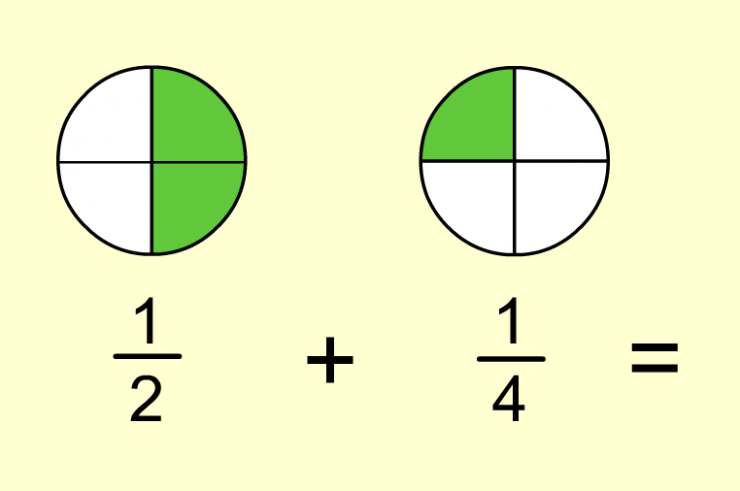Set 1 (10 Questions)
Add and subtract simple fractions (e.g., determine that 1/8+3/8 is the same as 1/2).
From Mr. Anker Tests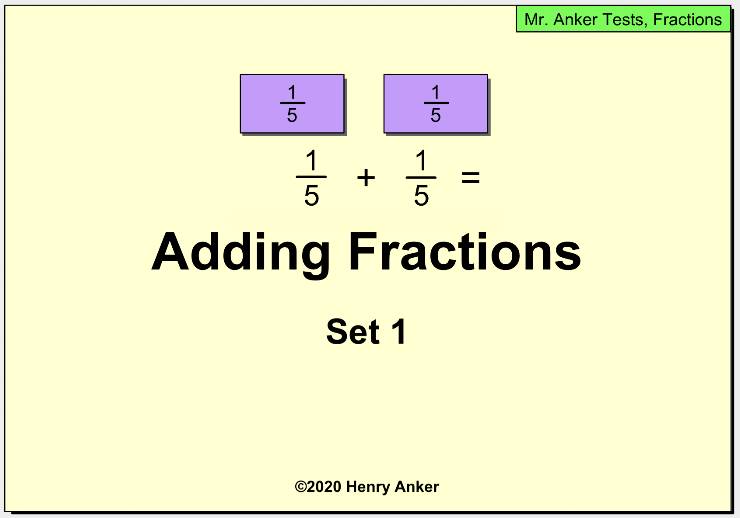1. What is the sum of 1/4 + 1/4? *
1 point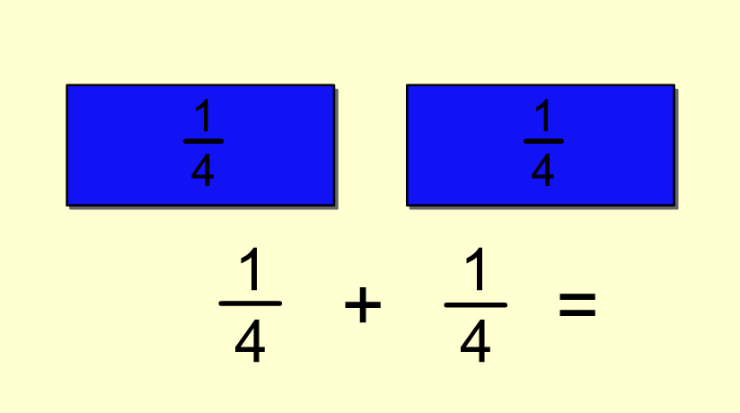2. What is the sum of 1/5 + 1/5? *
1 point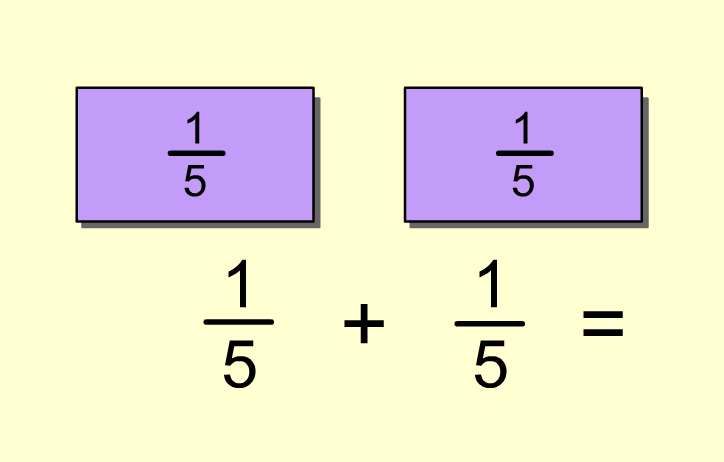3. What is the sum of 1/8 + 2/8? *
1 point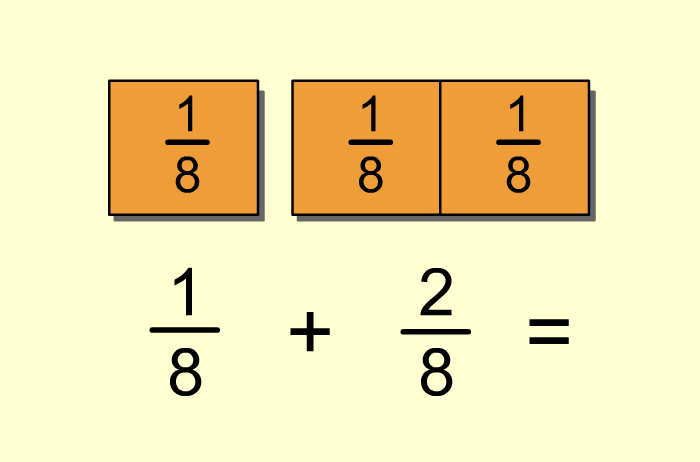4. What is the sum of 1/3 + 1/3? *
1 point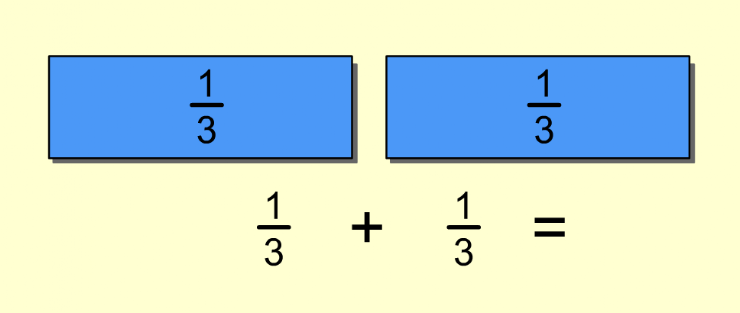5. What is the sum of 1/4 + 2/4? *
1 point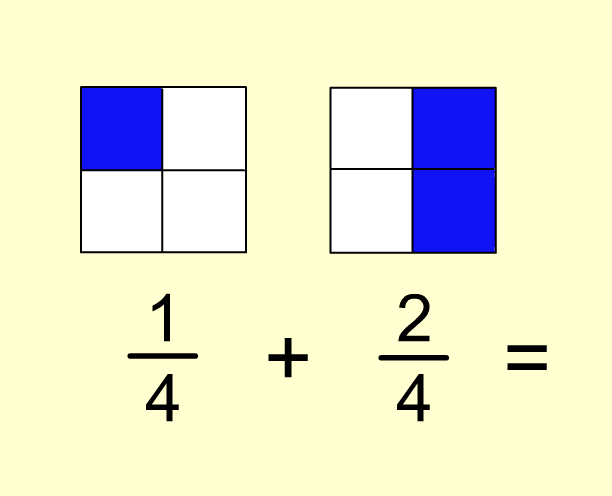6. What is the sum of 3/10 + 5/10? *
1 point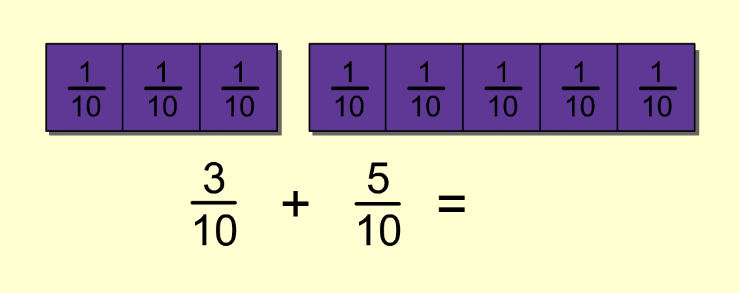7. What is the sum of 1/6+ 0/6 ? *
1 point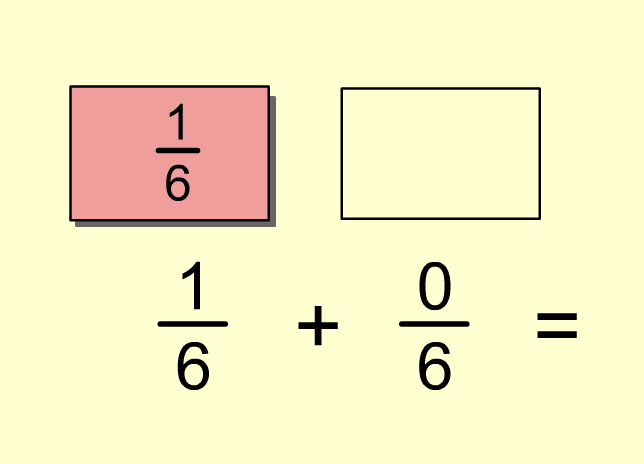8. What is the sum of 5/16+ 7/16? *
1 point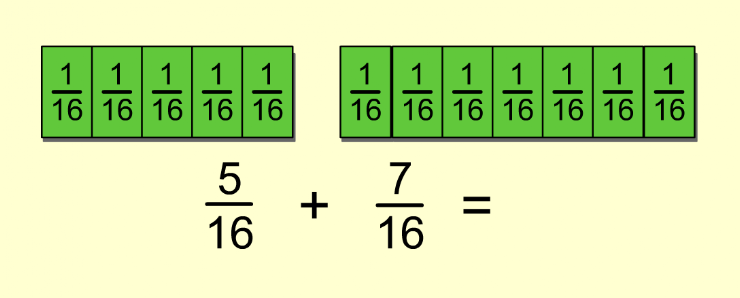8. What is the sum of 3/9+ 6/9? *
1 point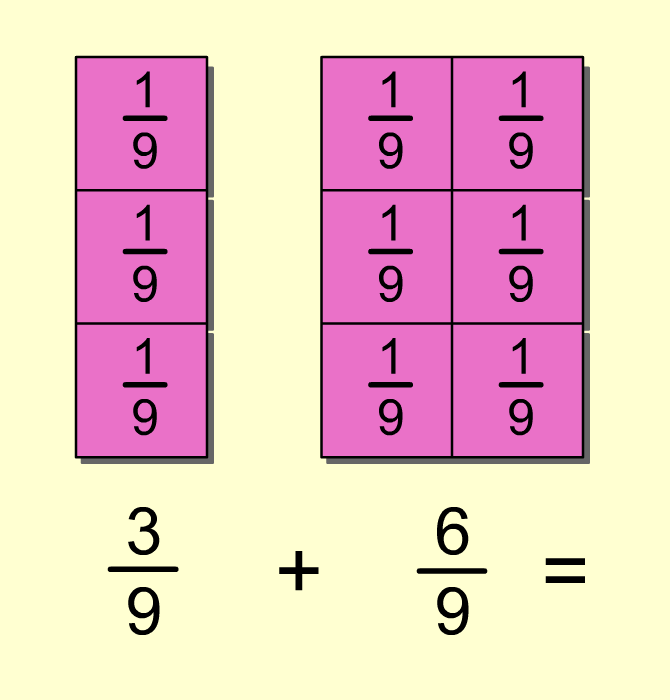10. What is the sum of 1/2+ 1/4? *
1 point